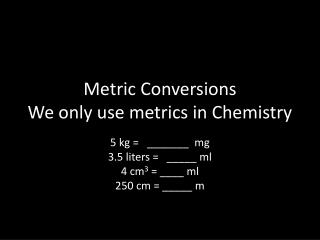Download PresentationMetric Conversions We only use metrics in Chemistry

# Metric Conversions We only use metrics in Chemistry

Download Presentation## Metric Conversions We only use metrics in Chemistry

- - - - - - - - - - - - - - - - - - - - - - - - - - - E N D - - - - - - - - - - - - - - - - - - - - - - - - - - -
##### Presentation Transcript

1. Metric ConversionsWe only use metrics in Chemistry 5 kg = _______ mg 3.5 liters = _____ ml 4 cm3 = ____ ml 250 cm = _____ m

2. The Metric System Compared to the English System: Mass = ? Volume = ? Length = ?

3. Metric is based on decimals. • Converting English units involves number changes: miles to inches. • Metric conversions simply involve moving the decimal point ( a power of ten). • Which way you move the decimal depends on whether going from a larger unit (Kg) to smaller (g) or the opposite. • Big to small – move it ?? • Small to big – move it ??

4. Ok, the size of units is represented by a set of prefixes! • Kilo = 1000 of anything. That’s 3 decimal place moves because of 3 zeros. • Centi = .01, that’s 1/100th of something; like 1 penny is .01 of a dollar. 1cent = \$0.01. That’s 2 decimal place moves. • Milli = .001, that’s 1/1000th of something; like 1 mm = .001 of a meter. 3 decimal moves.

5. What are the Base units in Metric? • Length: meter • Volume: liter • Mass: gram • Now, you can have a Kilogram (kg), a Kilometer (km) and a kiloliter (Kl). • You can have a centigram (cg) a centimeter (cm) and a centiliter (cl) • You can have a milligram (mg), a millimeter (mm) and a milliliter (ml).

6. Kilo  base unit centimilli 3 places 2 1 • So, from Kilograms to grams, you move the decimal 3 places to the right. • From Kg’s to milligrams, you move it 6 places to the right. • From milligrams to grams, you move the decimal 3 places to the left! • Then, 5 kilograms = _____ mg ? • And it works the same for all conversions !!!

7. Remember • Use the conversion for a while, but learn how to covert metric units without it. • When converting metric problems, only the decimal changes, NEVER the actual number. • 4.2 kg = 4200 grams.

8. Try These • Mass: 6.5 kg = ___ g 0.5 Kg = ___ g 150 kg = ___ g • 550 g = ____ kg 1250 g = ___ kg 0.5 g = ___ kg • Volume: 5.5 liters(l) = ___ ml 1750 L = ___ ml 0.45 L = __ ml 250 ml = ___ L 1500 ml = ___ L 750 ml = ___ L • Length: 4 km = ___ m 2.5 km = ___m 0.75 km = ___ m 2500 m = ___ km 500 m = ___ km 6 m = ___ cm 0.40 m = ___ cm 250 cm = ___ m 75 cm = ___ m 5 m = ___ mm 800 mm = ___ m * 7.5 km = ___ cm 5.5 km = ___ mm

9. Now, let’s try these !